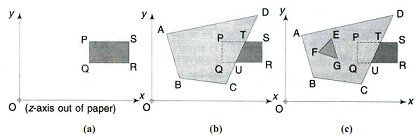## Determine in brief the painter algorithm, Data Structure & Algorithms

Assignment Help:

Determine in brief the Painter Algorithma) The farthest polygon, namely the rectangle PQRS, is stored first.

(b) The next farthest, the quadrilateral ABCD, is superposed, covering the  portion PQUT of the rectangle and leaving visible the portion TURS of the rectangle.

(c) Finally the closest, namely the triangle EFG, is superposed, entirely within the quadrilateral, and covering it in the region EFG.

The primary requirement for this algorithm is the sorting of polygons by priority, depending on whether a polygon covers (or is covered by) another polygon partly or wholly. This determination is made by comparing the x-, y-, and z-extents of the various polygons.

In case the z-extents of any two polygons overlap, then, if the two polygon planes are parallel to each other there is obscuring of one by the other; if the two polygon planes are not parallel, they may intersect each other. In such cases, the covered/uncovered or penetrated invisible/visible portions are determined by geometrical and clipping procedures, including the determination of the line of intersection of the two polygons if necessary, and separated into independent regions.

#### Representation of max-heap sequentially, Q. How do we represent a max-heap ...

Q. How do we represent a max-heap sequentially? Explain by taking a valid   example.         Ans: A max heap is also called as a descending heap, of size n is an almos

#### Algorithm to add an element at the end of linked list, Write an algorithm t...

Write an algorithm to add an element at the end of circular linked list.   Algorithm to Add the Element at the End of Circular Linked List. IINSENDCLL( INFO, LINK, START, A

#### Balance theorem, Question 1 Discuss the following theorems with respect to...

Question 1 Discuss the following theorems with respect to Splay Trees- Balance Theorem Dynamic Finger Theorem   Question 2 Write a C program for implementation

#### One dimenjsional array, how do you declare char text

how do you declare char text

#### Best case, for i=1 to n if a[i}>7 for j=2 to n a[j]=a{j}+j for n=2 to n a...

for i=1 to n if a[i}>7 for j=2 to n a[j]=a{j}+j for n=2 to n a[k]=a[j]+i else if a>4 && a for 2 to a a[j]= a{j]+5 else for 2to n a[j]=a[j]+i ..

#### #titlestrings, given the string "Data Structures & , Algorithms", write a p...

given the string "Data Structures & , Algorithms", write a program that uses sequential search to return index of ''&''

#### Link list, algorithm for multiplication of two sparse matrices using link l...

algorithm for multiplication of two sparse matrices using link list

#### Polynomials - represented by using arrays, /* the program accepts two polyn...

/* the program accepts two polynomials as a input & prints the resultant polynomial because of the addition of input polynomials*/ #include void main() { int poly1[

#### Multiple stacks in a single array, implement multiple stacks in an array an...

implement multiple stacks in an array and write different algorithms to perform operations on it

#### Explain the sum of subset problem, a. Explain the sum of subset problem. Ap...

a. Explain the sum of subset problem. Apply backtracking to solve the following instance of sum of subset problem: w= (3, 4, 5, 6} and d = 13. Briefly define the method using a sta